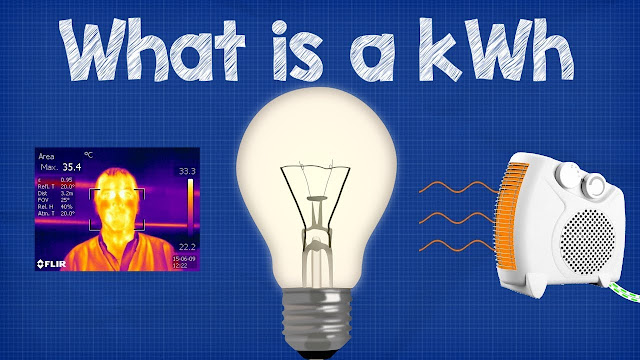What is a kWh - kilowatt hour + CALCULATIONS energy bill

# What is a kWh - kilowatt hour + CALCULATIONS energy billWhat is a kWh - kilowatt hour + CALCULATIONS energy bill

he kilowatt-hour (SI symbol: kW⋅h or kW h; commonly written as kWh) is a unit of energy equal to 3600 kilojoules (3.6 megajoules). The kilowatt-hour is commonly used as a billing unit for energy delivered to consumers by electric utilities.

#### Definition

The kilowatt-hour is a composite unit of energy equal to one kilowatt (kW) of power sustained for one hour. Expressed in the standard unit of energy in the International System of Units (SI), the joule (symbol J), it is equal to 3600 kilojoules (3.6 MJ).

The hour is a unit of time listed among the non-SI units accepted by the International Bureau of Weights and Measures for use with the SI. Its combination with the kilowatt, a standard SI unit, is therefore permitted within the standard.

#### Unit representations

A widely used symbolic representation of the kilowatt-hour is "kWh", from the unit symbols of its component units, kilowatt and hour. It is commonly used in commercial, educational, and scientific publications and in the media. It is also the usual unit representation in electrical power engineering. This common representation does not comply with the style guide of the International System of Units (SI).

Other representations of the unit may be encountered:
"kW⋅h" and "kW h" are less commonly used, but they are consistent with the SI. The SI brochure states that in forming a compound unit symbol, "Multiplication must be indicated by a space or a half-high (centered) dot (⋅), since otherwise some prefixes could be misinterpreted as a unit symbol." This is supported by a voluntary standard issued jointly by an international (IEEE) and national (ASTM) organization, and by a major style guide. However, the IEEE/ASTM standard allows "kWh" (but does not mention other multiples of the watt-hour). One guide published by NIST specifically recommends against "kWh" "to avoid possible confusion".
The United States official fuel-economy window sticker for electric vehicles uses the abbreviation "kW-hrs".

Variations in capitalization are sometimes encountered: KWh, KWH, kwh, etc., which are inconsistent with the International System of Units.
The notation "kW/h" for the kilowatt-hour is incorrect, as it denotes kilowatt per hour.
Electricity sales

Electrical energy is typically sold to consumers in kilowatt-hours. The cost of running an electrical device is calculated by multiplying the device's power consumption in kilowatts by the operating time in hours, and by the price per kilowatt-hour. The unit price of electricity charged by utility companies may depend on the customer's consumption profile over time. Prices vary considerably by locality. In the United States prices in different states can vary by a factor of three.

While smaller customer loads are usually billed only for energy, transmission services, and the rated capacity, larger consumers also pay for peak power consumption, the greatest power recorded in a fairly short time, such as 15 minutes. This compensates the power company for maintaining the infrastructure needed to provide peak power. These charges are billed as demand changes. Industrial users may also have extra charges according to the power factor of their load.

Major energy production or consumption is often expressed as terawatt-hours (TWh) for a given period that is often a calendar year or financial year. A 365-day year equals 8,760 hours, so over a period of one year, power of one gigawatt equates to 8.76 terawatt-hours of energy. Conversely, one terawatt-hour is equal to a sustained power of about 114 megawatts for a period of one year.

#### Examples

An electric heater consuming 1000 watts (1 kilowatt), and operating for one hour uses one kilowatt-hour of energy. A television consuming 100 watts operating for 10 hours continuously uses one kilowatt-hour. A 40-watt electric appliance operating continuously for 25 hours uses one kilowatt-hour. In terms of human power, a healthy adult male manual laborer performs work equal to about one half of one kilowatt-hour over an eight-hour day.

In this video we learn what a kWh is, the kilowatt hour is used for energy bills and related to the amount of electricity consumed by electrical items. We run through what a kilowatt and a kilowatt hour is as well as watts and joules. We see worked examples at the end for how to calculate kwh consumption and electricity bills.

Related topics:

Continue Reading about the "What is a kWh - kilowatt hour + CALCULATIONS energy bill" on the next page below